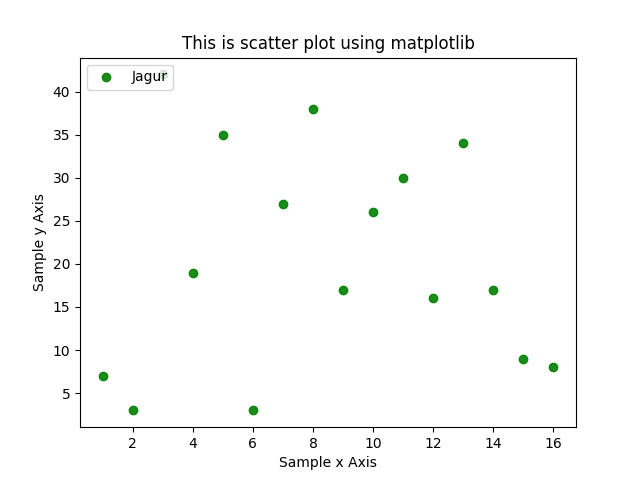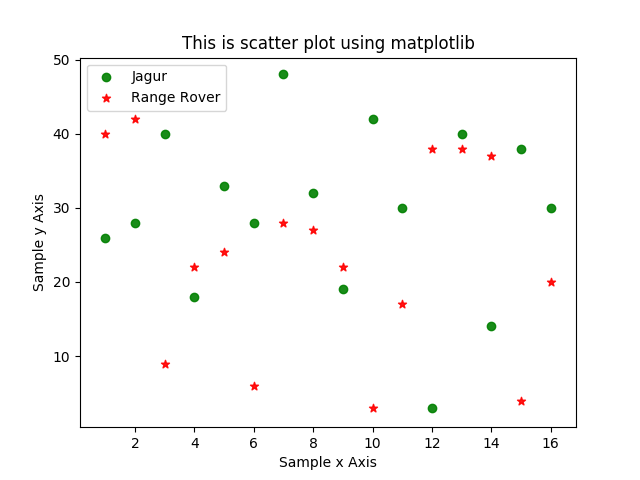# Scatter plots in Matplotlib – Data Visualization using Python

Scatter plots are one of the basic graphs used for data visualization. Scatter plot has following options, only first two are mandatory, rest all are optional.

plt.scatter(x, y, s=None, c=None, marker=None, cmap=None, norm=None, vmin=None, vmax=None, alpha=None, linewidths=None, verts=None, edgecolors=None, hold=None, data=None, **kwargs)

Here is simple program using scatter plot

Output of this program is as belowHere is a bit complex scatter plot using matplotlib

Output of above program